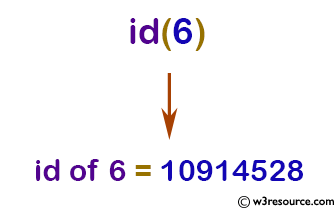# Python: input() function

## input() function

The input() function allows user input. If the prompt argument is present, it is written to standard output without a trailing newline.

The function then reads a line from input, converts it to a string (stripping a trailing newline), and returns that. When EOF is read, EOFError is raised.

Version:

(Python 3.2.5)

Syntax:

```input([prompt])
```

Parameter:

Name Description Required /
Optional
prompt A string that is written to standard output without trailing newline. Optional

Example: Python input() function

``````inputStr = input('Enter a string:')

print('The inputted string is:', inputStr)
``````

Output:

```Enter a string: Python input() Example
The inputted string is: Python input() Example
```

Pictorial Presentation:Python Code Editor:

Previous: id()
Next: int()

Test your Python skills with w3resource's quiz

﻿

## Python: Tips of the Day

How do I check if a list is empty?

For example, if passed the following:

```a = []
if not a:
print("List is empty")
```

Ref: https://bit.ly/2A4JXx9